EQUILIBRIUM CONSTANTS: Kc

```
```

This page explains what is meant by an equilibrium constant, introducing equilibrium constants expressed in terms of concentrations, Kc. It assumes that you are familiar with the concept of a dynamic equilibrium, and know what is meant by the terms "homogeneous" and "heterogeneous" as applied to chemical reactions.

Important:  If you aren't sure about dynamic equilibria it is important that you follow this link before you go on.

If you aren't sure what homogeneous and heterogeneous mean, you would find it useful to follow this link and read the beginning of the page that you will find (actually on catalysis).

We need to look at two different types of equilibria (homogeneous and heterogeneous) separately, because the equilibrium constants are defined differently.

• A homogeneous equilibrium has everything present in the same phase. The usual examples include reactions where everything is a gas, or everything is present in the same solution.

• A heterogeneous equilibrium has things present in more than one phase. The usual examples include reactions involving solids and gases, or solids and liquids.

```
```

Kc in homogeneous equilibria

This is the more straightforward case. It applies where everything in the equilibrium mixture is present as a gas, or everything is present in the same solution.

A good example of a gaseous homogeneous equilibrium is the conversion of sulphur dioxide to sulphur trioxide at the heart of the Contact Process: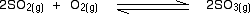A commonly used liquid example is the esterification reaction between an organic acid and an alcohol - for example: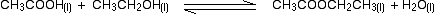```
```

Writing an expression for Kc

We are going to look at a general case with the equation:No state symbols have been given, but they will be all (g), or all (l), or all (aq) if the reaction was between substances in solution in water.

If you allow this reaction to reach equilibrium and then measure the equilibrium concentrations of everything, you can combine these concentrations into an expression known as an equilibrium constant.

The equilibrium constant always has the same value (provided you don't change the temperature), irrespective of the amounts of A, B, C and D you started with. It is also unaffected by a change in pressure or whether or not you are using a catalyst.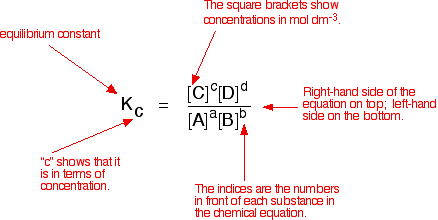Compare this with the chemical equation for the equilibrium. The convention is that the substances on the right-hand side of the equation are written at the top of the Kc expression, and those on the left-hand side at the bottom.

The indices (the powers that you have to raise the concentrations to - for example, squared or cubed or whatever) are just the numbers that appear in the equation.

Note:  If you have come across orders of reaction, don't confuse this with the powers that appear in the rate equation for a reaction. Those powers (the order of the reaction with respect to each of the reactants) are experimentally determined. They don't necessarily have any direct connection with the numbers that appear in the equation.

You may come across attempts to derive the expression for Kc by writing rate equations for the forward and back reactions. These attempts make the fundamental mistake of obtaining the rate equation from the chemical equation. That's WRONG! You could only do that for the very simple cases where the reaction took place in a single step represented by the given equation.

Georgio Karam contacted me in July 2015 pointing out that you can in fact derive the Kc expression for more complicated reactions by looking at each step of the reaction individually and then combining the results. You will find his article by following this link. If you know about orders of reaction and rate equations, you will find this very easy to follow.

The fact that this is possible doesn't, however, seem to me to justify using the derivation in general terms of aA plus bB going to cC and dD, and assuming that the numbers in front of the substances in the overall equation represent orders of reaction. That is dangerously misleading.

Some specific examples

The esterification reaction equilibrium

A typical equation might be:There is only one molecule of everything shown in the equation. That means that all the powers in the equilibrium constant expression are "1". You don't need to write those into the Kc expression.As long as you keep the temperature the same, whatever proportions of acid and alcohol you mix together, once equilibrium is reached, Kc always has the same value. At room temperature, this value is approximately 4 for this reaction.

The equilibrium in the hydrolysis of esters

This is the reverse of the last reaction: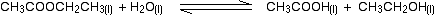The Kc expression is:```
```

If you compare this with the previous example, you will see that all that has happened is that the expression has turned upside-down. Its value at room temperature will be approximately 1/4 (0.25).

It is really important to write down the equilibrium reaction whenever you talk about an equilibrium constant. That is the only way that you can be sure that you have got the expression the right way up - with the right-hand substances on the top and the left-hand ones at the bottom.

The Contact Process equilibrium

You will remember that the equation for this is:This time the Kc expression will include some visible powers: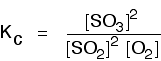```
```

Although everything is present as a gas, you still measure concentrations in mol dm-3. There is another equilibrium constant called Kp which is more frequently used for gases. You will find a link to that at the bottom of the page.

The Haber Process equilibrium

The equation for this is: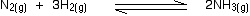. . . and the Kc expression is: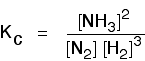```
```

Kc in heterogeneous equilibria

Typical examples of a heterogeneous equilibrium include:

The equilibrium established if steam is in contact with red hot carbon. Here we have gases in contact with a solid.If you shake copper with silver nitrate solution, you get this equilibrium involving solids and aqueous ions: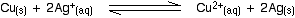```
```

Writing an expression for Kc for a heterogeneous equilibrium

The important difference this time is that you don't include any term for a solid in the equilibrium expression.

Taking another look at the two examples above, and adding a third one:

The equilibrium produced on heating carbon with steamEverything is exactly the same as before in the equilibrium constant expression, except that you leave out the solid carbon.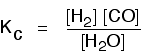```
```

The equilibrium produced between copper and silver ionsBoth the copper on the left-hand side and the silver on the right are solids. Both are left out of the equilibrium constant expression.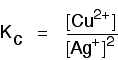```
```

The equilibrium produced on heating calcium carbonate

This equilibrium is only established if the calcium carbonate is heated in a closed system, preventing the carbon dioxide from escaping.The only thing in this equilibrium which isn't a solid is the carbon dioxide. That is all that is left in the equilibrium constant expression.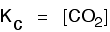```
```

Calculations involving Kc

There are all sorts of calculations you might be expected to do which are centred around equilibrium constants. You might be expected to calculate a value for Kc including its units (which vary from case to case). Alternatively you might have to calculate equilibrium concentrations from a given value of Kc and given starting concentrations.

This is simply too huge a topic to be able to deal with satisfactorily on the internet. It isn't the best medium for learning how to do chemistry calculations. It is much easier to do this from a carefully structured book giving you lots of worked examples and lots of problems to try yourself.

If you have found this site useful, you might like to have a look at my book on chemistry calculations. It covers equilibrium constant calculations starting with the most trivial cases, and gradually getting harder - up to the moderately difficult examples which may be asked in a UK A' level examination.

Note:  If you are interested in my chemistry calculations book you might like to follow this link.

```
```
 Questions to test your understanding If this is the first set of questions you have done, please read the introductory page before you start. You will need to use the BACK BUTTON on your browser to come back here afterwards. questions on Kc answers
```
```

Where would you like to go now?

To look at Kp . . .

To the equilibrium menu . . .

To the Physical Chemistry menu . . .

To Main Menu . . .

```
```